# Sets- Case Based Type Questions Notes | Study Mathematics (Maths) Class 11 - JEE

## JEE: Sets- Case Based Type Questions Notes | Study Mathematics (Maths) Class 11 - JEE

The document Sets- Case Based Type Questions Notes | Study Mathematics (Maths) Class 11 - JEE is a part of the JEE Course Mathematics (Maths) Class 11.
All you need of JEE at this link: JEE

Direction: The given table shows the 10 most famous engineering private colleges of India with their respective fee structure of 4 years Bachelor of Technology course.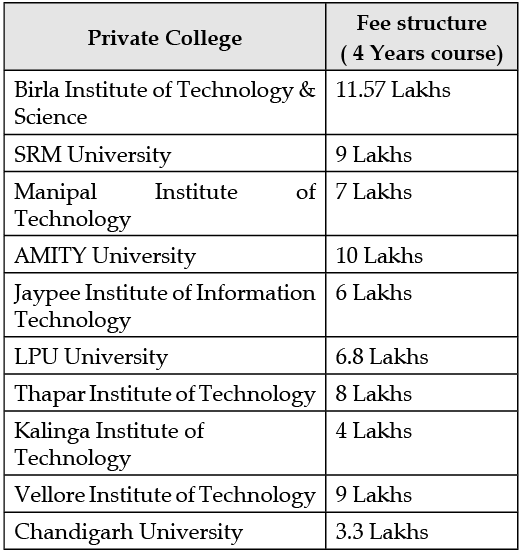Question 1:
Ram wants to take admission in private engineering but has a budget of 6 lakhs. Find the set of colleges in which Ram takes the admission.
(a) {SRM, Birla, LPU, Chandigarh, Kalinga}
(b) {Jaypee, LPU, Chandigarh, Kalinga}
(c) {Jaypee, Chandigarh, Kalinga}
(d) {AMITY, Vellore, Chandigarh, Kalinga}

From the table, it is observed that there are 3 colleges whose fees for 4 year course is less than or equal to 6 lakhs. So, the required set is {Jaypee, Chandigarh, Kalinga}.

Question 2:
If Sri and Ram wants to take admission in the same college. Find the set of college in which both Sri and Ram can take their admission.

(a) {Chandigarh, Kalinga}
(b) {Jaypee}
(c) {SRM, LPU}
(d) Ø

The common colleges in which Sri and Ram both of them can take admission are Chandigarh and Kalinga. So, the required set is {Chandigarh, Kalinga}.

Question 3:
Sri has taken an education loan of 3 lakhs to increase his overall budget. Find the set of college in which Ram can take admission but Sri cannot.
(a) {Birla, Thapar}
(b) {Thapar}
(c) {SRM}
(d) {Manipal, Thapar}

On increasing the budget of Sri by 3 lakh, the total budget will be 7 lakhs. So, the set of college in which she can take admission are {Manipal, LPU, Jaypee, Chandigarh, Kalinga}. But the set of colleges in which Ram can take admission are {Manipal, LPU, Thapar, Jaypee, Chandigarh, Kalinga}. Since there is one element that is not common in both the sets, the required set is {Thapar}.

Question 4:
Sri has a total budget of 4 lakhs and she wanted  admission in the famous college. Will she able to take admission in the colleges given in table. Find the set of college in which she can take admission.
(a) Yes, {SRM, Birla, LPU}
(b) No, {Jaypee, LPU, Kalinga}
(c) Yes, {Chandigarh, Kalinga}
(d) None of the above

Yes, there are two colleges whose fees are either equal to or less than 4 lakhs. So, the required set is {Chandigarh, Kalinga}.

Question 5:
Ram has taken an education loan of 2 lakhs to increase his overall budget. Find the number of college in which he can take admission after raising his budget.
(a) 4
(b) 6
(c) 7
(d) 9

Earlier when the budget was 6 lakh, the set of colleges are {Jaypee, Chandigarh, Kalinga}. On increasing the budget by 2 lakhs, the total budget of Ram becomes 8 lakhs. So, the set of college in which he can take admission are {Manipal, LPU, Thapar, Jaypee, Chandigarh, Kalinga}.

Direction: A survey is conducted by a career counsellor in a college to find career choice of students after the Intermediate. There are 100 students that goes for Engineering Courses, 50 wants to make their career in Medical, 100 students continue their further study in Arts. There are 10 students that go for both Engineering and Medical, and 3 goes for Medical and Arts. There are 3 students that do not go for any further studies.
Question 6:
Find the total number of students on which the survey is conducted.
(a) 250
(b) 230
(c) 240
(d) 238

Let A set denotes Engineering, B set denotes Medical and C set denotes Art. Draw the Venn’s diagram for the given situation.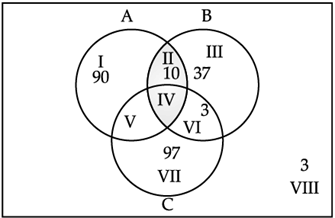Total number of students is the sum of elements in all the regions. So, the total number of students are 240.

Question 7:
Find the number of students that goes for both Medical and Art.
(a) 5
(b) 2
(c) 3
(d) 4

Let A set denotes Engineering, B set Medical and C set Art. Draw the Venn’s diagram for the given situation.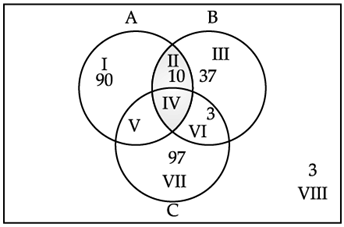The region VI shows the student that goes for both Medical and Arts. So, n(B ∩ C) = 3.

Question 8:
Find the number of students that goes for only engineering course.
(a) 90
(b) 95
(c) 100
(d) 93

Let A set denotes Engineering, B set denotes Medical and C set denotes Art. Draw the Venn’s diagram for the given situation.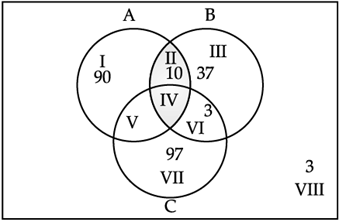So, the number of students that goes for only engineering course are 90.

Question 9:
Find the number of students that goes for medical or engineering.
(a) 150
(b) 140
(c) 160
(d) No of the above

Let A set denotes Engineering, B set denotes Medical and C set denotes Art. Draw the Venn’s diagram for the given situation.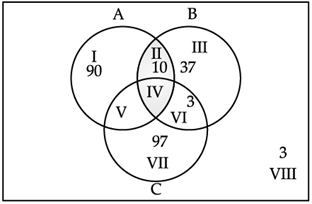The region I, II, III, IV, V, and VI shows the student that goes for Engineering or Medical. So, the required number of students is 140.

Question 10:
Find the number of students that goes for Engineering or Art.
(a) 150
(b) 200
(c) 190
(d) 197

Let A set denotes Engineering, B set denotes Medical and C set denotes Art. Draw the Venn’s diagram for the given situation.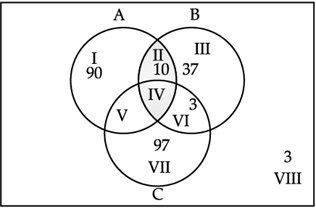The region I, II, IV, IV, V, and VII shows the student that goes for Engineering or Arts. So, the required number of students is 200.

The document Sets- Case Based Type Questions Notes | Study Mathematics (Maths) Class 11 - JEE is a part of the JEE Course Mathematics (Maths) Class 11.
All you need of JEE at this link: JEEUse Code STAYHOME200 and get INR 200 additional OFF

## Mathematics (Maths) Class 11

154 videos|171 docs|132 tests

Track your progress, build streaks, highlight & save important lessons and more!

,

,

,

,

,

,

,

,

,

,

,

,

,

,

,

,

,

,

,

,

,

;